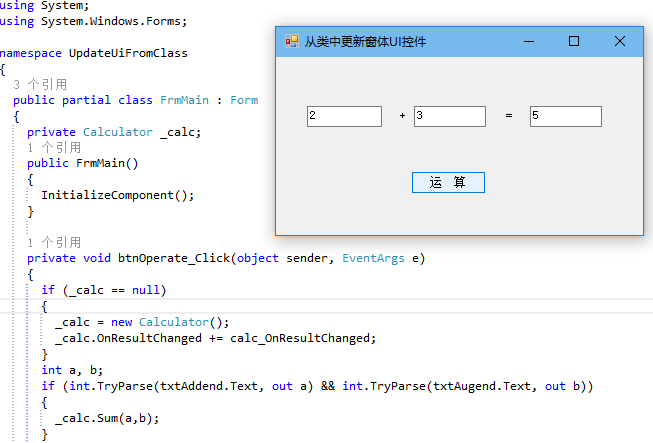# C#/.NET WINFORM中使用委托和事件在类中更新窗体UI控件

55342 发布于: 2015-08-29 09:05 读完约需 2 分钟

``````namespace UpdateUiFromClass
{
//声明一个计算器类
public class Calculator
{
/// <summary>
/// 声明一个监控结果变化的委托
/// </summary>
/// <param name="sender"></param>
/// <param name="e"></param>
public delegate void ResultChangedHandler(object sender, object e);
/// <summary>
/// 声明一个监控结果变化的事件
/// </summary>
public event ResultChangedHandler OnResultChanged;

private int _result;
/// <summary>
/// 计算器的计算结果
/// </summary>
public int Result
{
get { return _result; }
}

//处理注册的事件
protected virtual void RaiseResultChanged(object sender, object e)
{
var handler = OnResultChanged;
//判断是否注册了OnResultChanged事件，只有注册了OnResultChanged事件才执行事件对应的方法
if (handler != null) handler(sender, e);
}

/// <summary>
/// 加法操作
/// </summary>
/// <param name="a"></param>
/// <param name="b"></param>
public void Sum(int a, int b)
{
var temp = a + b;
if (_result == temp) return;
_result = temp;
RaiseResultChanged(this, _result);
}
}
}
``````

``````using System;
using System.Windows.Forms;

namespace UpdateUiFromClass
{
public partial class FrmMain : Form
{
/// <summary>
/// 私有的计算器变量
/// </summary>
private Calculator _calc;
public FrmMain()
{
InitializeComponent();
}

private void btnOperate_Click(object sender, EventArgs e)
{
if (_calc == null)
{
//实例化一个计算器
_calc = new Calculator();
//注册计算结果变化时要触发的事件
_calc.OnResultChanged += calc_OnResultChanged;
}
int a, b;
if (int.TryParse(txtAddend.Text, out a) && int.TryParse(txtAugend.Text, out b))
{
_calc.Sum(a,b);
}

}

/// <summary>
/// 计算结果变化时触发事件的方法
/// </summary>
/// <param name="sender"></param>
/// <param name="e"></param>
void calc_OnResultChanged(object sender, object e)
{
//此行用的是Calculator类中的Result属性值
//txtResult.Text = _calc.Result.ToString();

//此行用的是Calculator类中委托参数传入的值
txtResult.Text = e.ToString();

/*
*
* 以下是对sender参数的操作
* 你可以把注释取消来查看执行结果
*
*
//此行是获取被实例化后的Calculator类中委托传入的第一个参数，即sender
var calc = (Calculator) sender;

//获取到sender后，我们就可以对它进行相关的操作了，这里的sender就是上面声明的_calc实例
MessageBox.Show(calc.Result.ToString());
*
*/
}
}
}
``````